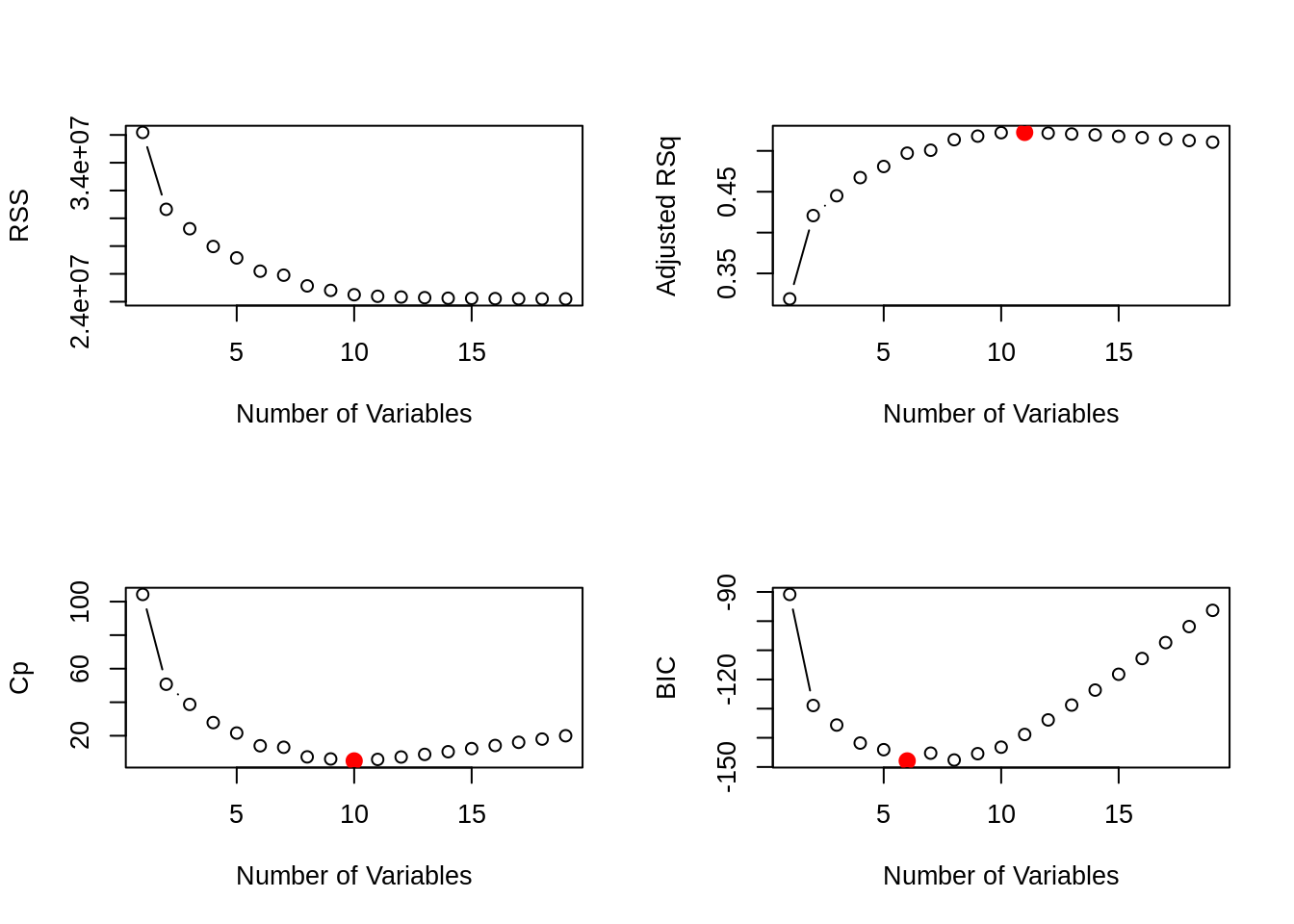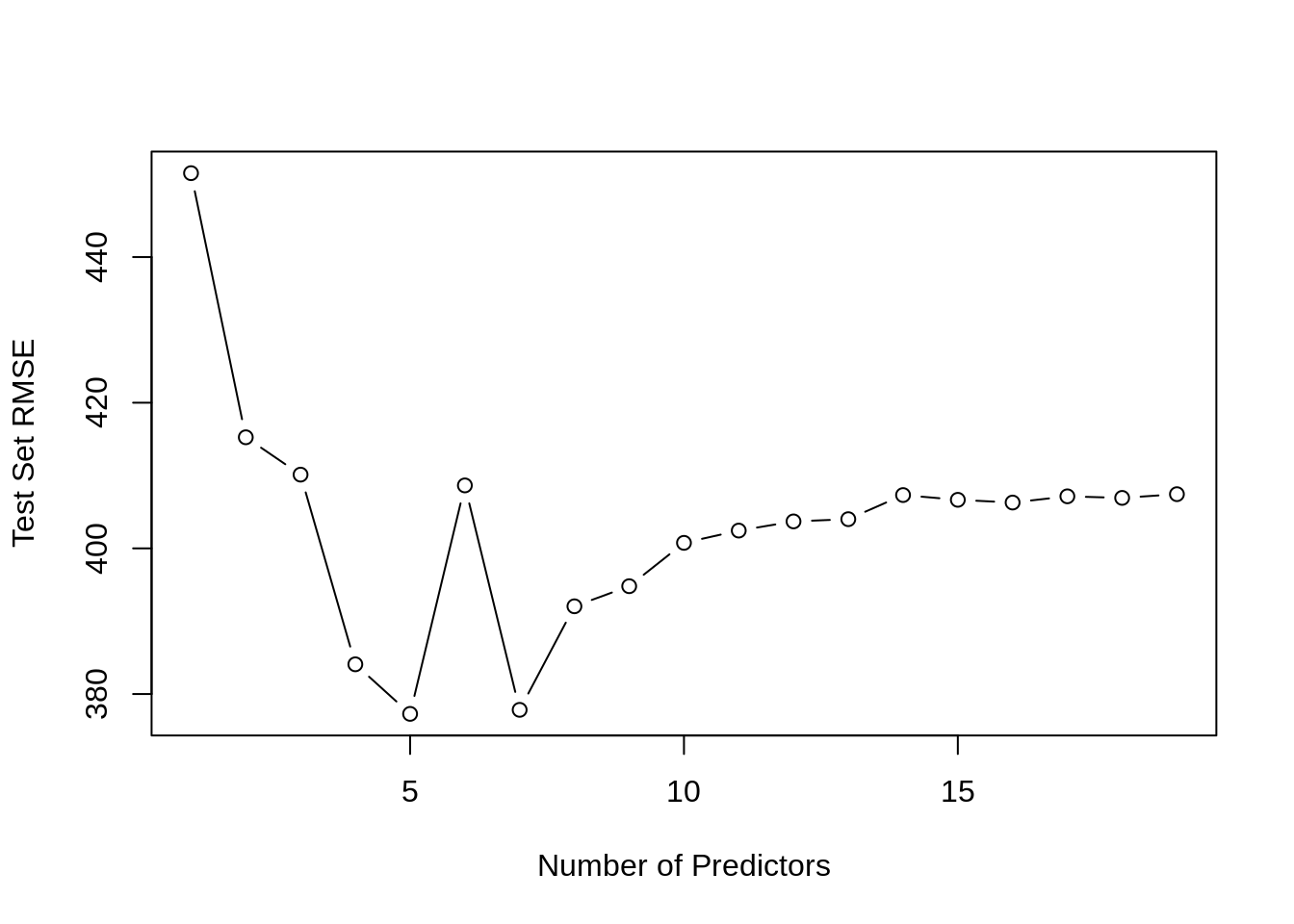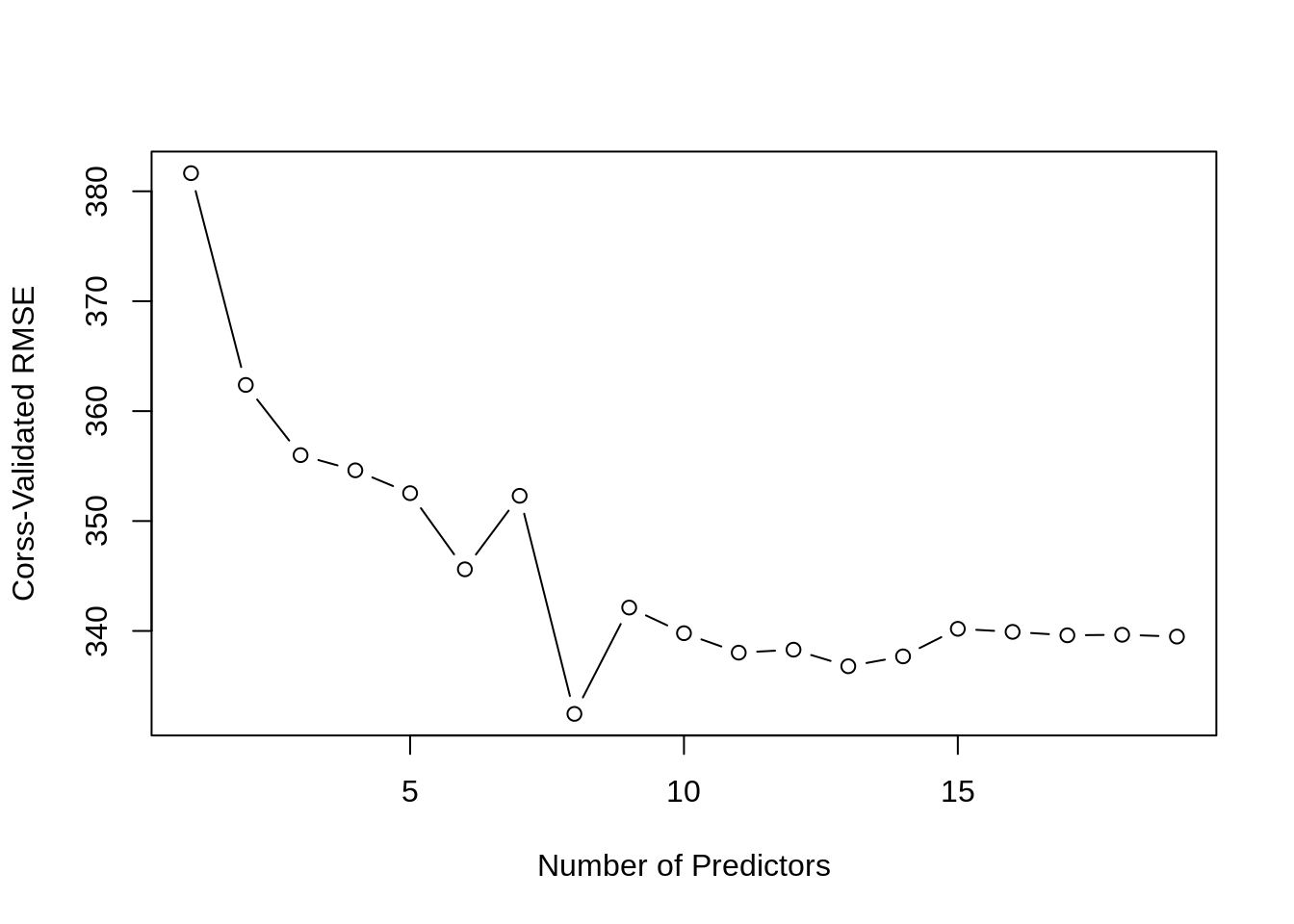# Chapter 22 Subset Selection

Instructor’s Note: This chapter is currently missing the usual narrative text. Hopefully it will be added later.

data(Hitters, package = "ISLR")
sum(is.na(Hitters))
##  59
sum(is.na(Hitters$Salary)) ##  59 Hitters = na.omit(Hitters) sum(is.na(Hitters)) ##  0 ## 22.1 AIC, BIC, and Cp ### 22.1.1leaps Package library(leaps) ### 22.1.2 Best Subset fit_all = regsubsets(Salary ~ ., Hitters) summary(fit_all) ## Subset selection object ## Call: regsubsets.formula(Salary ~ ., Hitters) ## 19 Variables (and intercept) ## Forced in Forced out ## AtBat FALSE FALSE ## Hits FALSE FALSE ## HmRun FALSE FALSE ## Runs FALSE FALSE ## RBI FALSE FALSE ## Walks FALSE FALSE ## Years FALSE FALSE ## CAtBat FALSE FALSE ## CHits FALSE FALSE ## CHmRun FALSE FALSE ## CRuns FALSE FALSE ## CRBI FALSE FALSE ## CWalks FALSE FALSE ## LeagueN FALSE FALSE ## DivisionW FALSE FALSE ## PutOuts FALSE FALSE ## Assists FALSE FALSE ## Errors FALSE FALSE ## NewLeagueN FALSE FALSE ## 1 subsets of each size up to 8 ## Selection Algorithm: exhaustive ## AtBat Hits HmRun Runs RBI Walks Years CAtBat CHits CHmRun CRuns CRBI ## 1 ( 1 ) " " " " " " " " " " " " " " " " " " " " " " "*" ## 2 ( 1 ) " " "*" " " " " " " " " " " " " " " " " " " "*" ## 3 ( 1 ) " " "*" " " " " " " " " " " " " " " " " " " "*" ## 4 ( 1 ) " " "*" " " " " " " " " " " " " " " " " " " "*" ## 5 ( 1 ) "*" "*" " " " " " " " " " " " " " " " " " " "*" ## 6 ( 1 ) "*" "*" " " " " " " "*" " " " " " " " " " " "*" ## 7 ( 1 ) " " "*" " " " " " " "*" " " "*" "*" "*" " " " " ## 8 ( 1 ) "*" "*" " " " " " " "*" " " " " " " "*" "*" " " ## CWalks LeagueN DivisionW PutOuts Assists Errors NewLeagueN ## 1 ( 1 ) " " " " " " " " " " " " " " ## 2 ( 1 ) " " " " " " " " " " " " " " ## 3 ( 1 ) " " " " " " "*" " " " " " " ## 4 ( 1 ) " " " " "*" "*" " " " " " " ## 5 ( 1 ) " " " " "*" "*" " " " " " " ## 6 ( 1 ) " " " " "*" "*" " " " " " " ## 7 ( 1 ) " " " " "*" "*" " " " " " " ## 8 ( 1 ) "*" " " "*" "*" " " " " " " fit_all = regsubsets(Salary ~ ., data = Hitters, nvmax = 19) fit_all_sum = summary(fit_all) names(fit_all_sum) ##  "which" "rsq" "rss" "adjr2" "cp" "bic" "outmat" "obj" fit_all_sum$bic
##    -90.84637 -128.92622 -135.62693 -141.80892 -144.07143 -147.91690
##   -145.25594 -147.61525 -145.44316 -143.21651 -138.86077 -133.87283
##  -128.77759 -123.64420 -118.21832 -112.81768 -107.35339 -101.86391
##   -96.30412
par(mfrow = c(2, 2))
plot(fit_all_sum$rss, xlab = "Number of Variables", ylab = "RSS", type = "b") plot(fit_all_sum$adjr2, xlab = "Number of Variables", ylab = "Adjusted RSq", type = "b")
best_adj_r2 = which.max(fit_all_sum$adjr2) points(best_adj_r2, fit_all_sum$adjr2[best_adj_r2],
col = "red",cex = 2, pch = 20)

plot(fit_all_sum$cp, xlab = "Number of Variables", ylab = "Cp", type = 'b') best_cp = which.min(fit_all_sum$cp)
points(best_cp, fit_all_sum$cp[best_cp], col = "red", cex = 2, pch = 20) plot(fit_all_sum$bic, xlab = "Number of Variables", ylab = "BIC", type = 'b')
best_bic = which.min(fit_all_sum$bic) points(best_bic, fit_all_sum$bic[best_bic],
col = "red", cex = 2, pch = 20)### 22.1.3 Stepwise Methods

fit_fwd = regsubsets(Salary ~ ., data = Hitters, nvmax = 19, method = "forward")
fit_fwd_sum = summary(fit_fwd)
fit_bwd = regsubsets(Salary ~ ., data = Hitters, nvmax = 19, method = "backward")
fit_bwd_sum = summary(fit_bwd)
coef(fit_fwd, 7)
##  (Intercept)        AtBat         Hits        Walks         CRBI       CWalks
##  109.7873062   -1.9588851    7.4498772    4.9131401    0.8537622   -0.3053070
##    DivisionW      PutOuts
## -127.1223928    0.2533404
coef(fit_bwd, 7)
##  (Intercept)        AtBat         Hits        Walks        CRuns       CWalks
##  105.6487488   -1.9762838    6.7574914    6.0558691    1.1293095   -0.7163346
##    DivisionW      PutOuts
## -116.1692169    0.3028847
coef(fit_all, 7)
##  (Intercept)         Hits        Walks       CAtBat        CHits       CHmRun
##   79.4509472    1.2833513    3.2274264   -0.3752350    1.4957073    1.4420538
##    DivisionW      PutOuts
## -129.9866432    0.2366813
fit_bwd_sum = summary(fit_bwd)
which.min(fit_bwd_sum$cp) ##  10 coef(fit_bwd, which.min(fit_bwd_sum$cp))
##  (Intercept)        AtBat         Hits        Walks       CAtBat        CRuns
##  162.5354420   -2.1686501    6.9180175    5.7732246   -0.1300798    1.4082490
##         CRBI       CWalks    DivisionW      PutOuts      Assists
##    0.7743122   -0.8308264 -112.3800575    0.2973726    0.2831680
fit = lm(Salary ~ ., data = Hitters)
fit_aic_back = step(fit, trace = FALSE)
coef(fit_aic_back)
##  (Intercept)        AtBat         Hits        Walks       CAtBat        CRuns
##  162.5354420   -2.1686501    6.9180175    5.7732246   -0.1300798    1.4082490
##         CRBI       CWalks    DivisionW      PutOuts      Assists
##    0.7743122   -0.8308264 -112.3800575    0.2973726    0.2831680

## 22.2 Validated RMSE

set.seed(42)
num_vars = ncol(Hitters) - 1
trn_idx = sample(c(TRUE, FALSE), nrow(Hitters), rep = TRUE)
tst_idx = (!trn_idx)

fit_all = regsubsets(Salary ~ ., data = Hitters[trn_idx, ], nvmax = num_vars)
test_mat = model.matrix(Salary ~ ., data = Hitters[tst_idx, ])

test_err = rep(0, times = num_vars)
for (i in seq_along(test_err)) {
coefs = coef(fit_all, id = i)
pred = test_mat[, names(coefs)] %*% coefs
test_err[i] <- sqrt(mean((Hitters$Salary[tst_idx] - pred) ^ 2)) } test_err ##  451.5144 415.2496 410.1213 384.0785 377.2735 408.6423 377.8263 392.0436 ##  394.8037 400.7555 402.4398 403.6991 404.0057 407.3175 406.6637 406.2891 ##  407.1439 406.9280 407.4385 plot(test_err, type='b', ylab = "Test Set RMSE", xlab = "Number of Predictors")which.min(test_err) ##  5 coef(fit_all, which.min(test_err)) ## (Intercept) Walks CAtBat CHits CHmRun PutOuts ## 102.0163457 3.7019974 -0.4002084 1.5041824 2.9007854 0.1975283 class(fit_all) ##  "regsubsets" predict.regsubsets = function(object, newdata, id, ...) { form = as.formula(object$call[])
mat   = model.matrix(form, newdata)
coefs = coef(object, id = id)
xvars = names(coefs)

mat[, xvars] %*% coefs
}
rmse = function(actual, predicted) {
sqrt(mean((actual - predicted) ^ 2))
}
num_folds = 5
num_vars  = 19
set.seed(1)
folds = caret::createFolds(Hitters$Salary, k = num_folds) fold_error = matrix(0, nrow = num_folds, ncol = num_vars, dimnames = list(paste(1:5), paste(1:19))) for(j in 1:num_folds) { train_fold = Hitters[-folds[[j]], ] validate_fold = Hitters[ folds[[j]], ] best_fit = regsubsets(Salary ~ ., data = train_fold, nvmax = 19) for (i in 1:num_vars) { pred = predict(best_fit, validate_fold, id = i) fold_error[j, i] = rmse(actual = validate_fold$Salary,
predicted = pred)
}

}

cv_error = apply(fold_error, 2, mean)
cv_error
##        1        2        3        4        5        6        7        8
## 381.6473 362.3809 355.9959 354.6139 352.5358 345.6078 352.2963 332.4575
##        9       10       11       12       13       14       15       16
## 342.1292 339.7967 338.0266 338.2973 336.7897 337.6876 340.1955 339.9188
##       17       18       19
## 339.6058 339.6544 339.4893
plot(cv_error, type='b', ylab = "Corss-Validated RMSE", xlab = "Number of Predictors")fit_all = regsubsets(Salary ~ ., data = Hitters, nvmax = num_vars)
coef(fit_all, which.min(cv_error))
##  (Intercept)        AtBat         Hits        Walks       CHmRun        CRuns
##  130.9691577   -2.1731903    7.3582935    6.0037597    1.2339718    0.9651349
##       CWalks    DivisionW      PutOuts
##   -0.8323788 -117.9657795    0.2908431

## 22.4 RMarkdown

The RMarkdown file for this chapter can be found here. The file was created using R version 4.0.2 and the following packages:

• Base Packages, Attached
##  "stats"     "graphics"  "grDevices" "utils"     "datasets"  "methods"
##  "base"
##  "leaps"
##   "tidyselect"   "xfun"         "purrr"        "reshape2"     "splines"
##  "compiler"